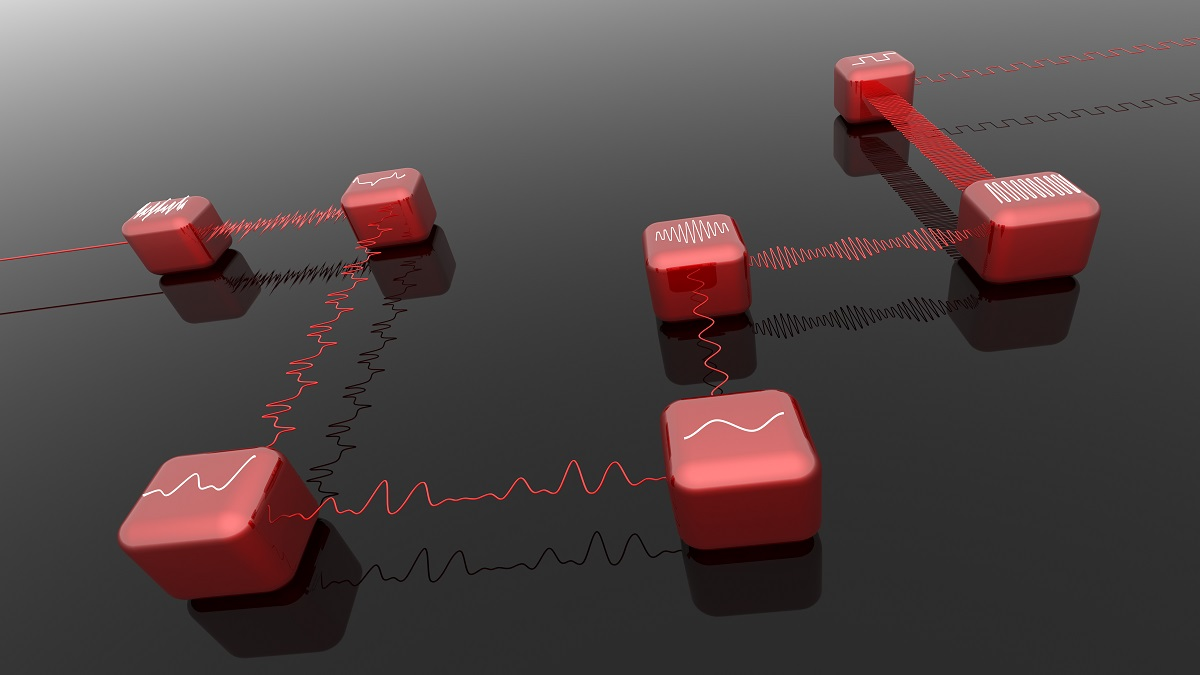# Optimize Signal Conditioning for EVM Measurements

Exponential growth in demand for faster data rate applications has triggered the need for technologies capable of wide signal bandwidth at higher frequencies. Unfortunately, wider bandwidths also gather more noise. Wideband noise and excess path loss at millimeter frequencies between signal analyzers and devices under test (DUTs) result in a lower signal-to-noise (SNR) for the digitizer. This post will address the impacts of SNR and how to optimize signal conditioning of a signal analyzer for accurate EVM measurements.

## Making EVM Measurements

Error vector magnitude (EVM) measurements provide a simple and quantitative figure of merit for a digital modulation signal. The errors may result from the phase noise of local oscillators (LOs), noise from power amplifiers, IQ modulator impairments, and many other sources. Figure 1 shows the modulation analysis used for common modulation formats. The IQ measured waveform data goes into a demodulator for recovering original data bits, then modulates the data bits to get the IQ reference (ideal) waveform. The other path is the IQ measured waveform data processed with signal compensation and a measurement filter. The signal error is the difference between the reference waveform and the compensated measured waveform.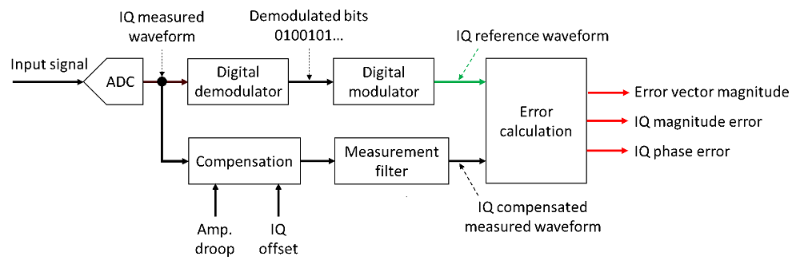Figure 1. Error vector signal analysis block diagram

Figure 2 illustrates the vector signal error. The error vector (red arrow) goes from the detected point of the IQ reference signal vector (green arrow) to the IQ measured signal vector (black arrow). EVM is the root mean square of the error vectors computed, expressed as a percentage of the EVM normalization reference.Figure 2. Illustration of EVM calculation

## Optimize SNR for Accurate EVM Measurements

Figure 3 is a simplified block diagram of a vector signal analyzer. The low SNR causes the transmitter measurements to have a poor EVM and adjacent channel power ratio (ACPR) performance, which does not represent the performance of the DUT, as shown in Figure 4. The noise power includes anything that causes the symbol to deviate from the ideal state position, including noise from the DUT and the signal analyzer. At mmWave frequencies, excess cable loss makes RF power limited and costly. You need to measure performance metrics using over-the-air (OTA) test methods at mmWave frequencies, making it more difficult to achieve accurate and repeatable results.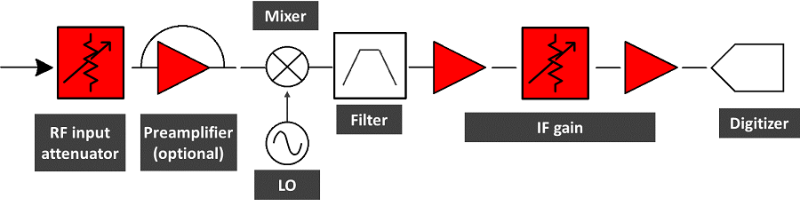Figure 3. A signal analyzer​ block diagram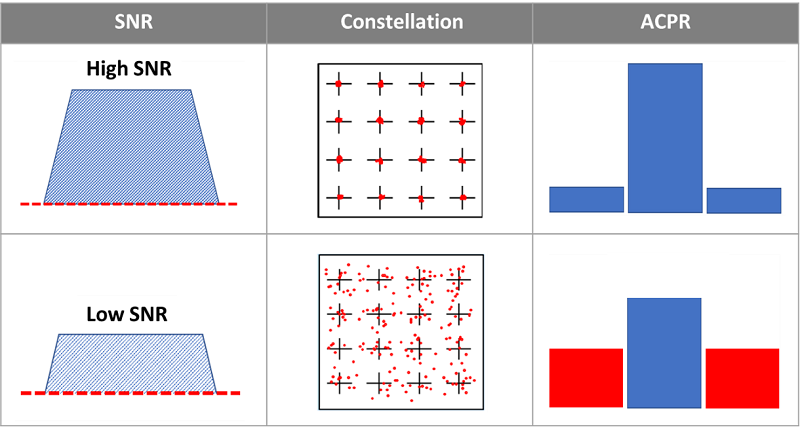Figure 4. Signal to noise ratio (SNR) impacts on transmitter measurements

The system intermediate frequency (IF) noise of a signal analyzer must be low enough to get the best EVM measurement results. On the other hand, the input signal to the digitizer must be high enough without overloading the digitizer. This requires a combination setup of RF attenuators, preamplifier, and IF gain value based on the measured signal peak level.

## Accelerate Signal Conditioning for EVM Measurements

New signal analyzers let you press a single key to optimize these hardware settings, improving SNR and avoiding digitizer overload, as shown in Figure 5. The optimization processing requires measuring the signal peak level and setting up the analyzer. However, the measured period may not represent the complete power characteristics of the input signal. A user can manually tweak the settings, such as IF gain and RF attenuators, to achieve the best measurement results.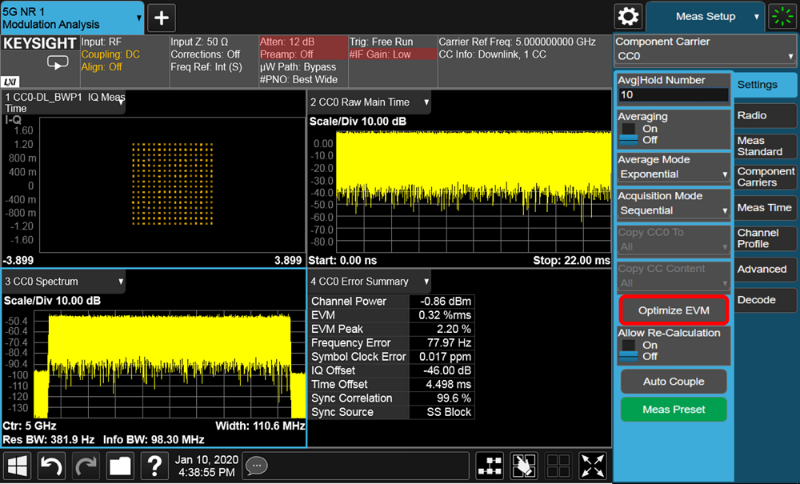Figure 5. Optimizing EVM measurements for 5G NR modulation analysis

See related posts to learn about how to optimize signal demodulation analysis:

limit
3